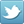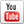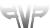User Name: Password: Remember Me?   Register for your free account! | Forgot your password?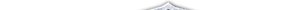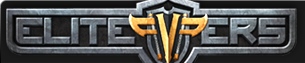You last visited: Today at 19:59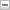•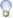Please register to post and access all features, it's quick, easy and FREE!# noob question

Discussion on noob question within the CO2 Private Server forum part of the Conquer Online 2 category.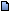02/17/2010, 00:56 #1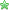elite*gold: 0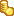The Black Market: 0/0/0 Join Date: Nov 2009 Posts: 18 Received Thanks: 2 noob question sorry for this noob question but im new and want to move some npcs to twin. *anyone knows all maps ids? *how can i disable pots like chantpill *how can i make pendlar twin npc sell pray obs: for 5165 source thx anyway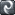02/17/2010, 00:58 #2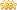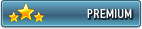elite*gold: 0The Black Market: 3/0/0 Join Date: Oct 2009 Posts: 8,740 Received Thanks: 5,276 #request move This is a guides/release section. If you have any questions pertaining CO2 private servers please post them here,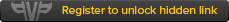02/17/2010, 01:10 #3elite*gold: 0The Black Market: 0/0/0 Join Date: Oct 2009 Posts: 63 Received Thanks: 10 those r da 1s i found Code: ```Desert = 1000, AncientMaze = 1001, //(MYSTIC CASTLE) TwinCity = 1002, Mine = 1003, Promotion = 1004, Arena = 1005, Stables = 1006, BirthVillage = 1010, PhoenixCastle = 1011, RebirthMap = 1012, HawkCave = 1013, BanditCave = 1014, BatCave = 1016, AdvanceZone = 1017, ApeMoutain = 1020, DesertCity = 1000, ReedIsland = 1015, //(BIRD ISLAND) DreamLand = 1012, KylinCave = 1016, TrainingGround = 1039, Market = 1036, Lottery = 700, Jail = 6000, GuildArea = 1038 grocery2 = 1009, mine01 = 1003, forum = 1004, horse = 1006, star01 = 1100, star02 = 1101, star03 = 1102, star04 = 1103, star05 = 1104, star10 = 1105, star06 = 1106, star07 = 1107, star08 = 1108, star09 = 1109, smith = 1007, ArmorColour = 1008, sky = 1012, warena = 1017,61,145(this 1) parena = 1018,89,10(GW) larena = 1019, mine = 1021, brave = 1022, mineone = 1025, minetwo = 1026, minethree = 1027, minefour = 1028, mineone2 = 1029, minetwo2 = 1030, minethree2 = 1031, minefour2 = 1032, newbie2 = 1035, playground = 1039, mineone3 = 5000, factionblack = 1037, skycut = 1040, skymaze = 1041, prison2 = 6001, lineuppass = 1042, lineup = 1043, lineup2 = 1044, lineup3 = 1045, lineup4 = 1046, lineup5 = 1047, lineup6 = 1048, lineup7 = 1049, lineup8 = 1050, riskisland = 1051, skymaze1 = 1060, skymaze2 = 1061, skymaze3 = 1062, star = 1064, boa = 1070, parena1 = 1080,(GW) parena2 = 1081,(GW) newcanyon = 1075, MapleForest = 1076, newdesert = 1077, newisland = 1078, mysisland = 1079, riskisland1 = 1063, idlandmap = 1082, parenam = 1090, parenas = 1091, house01 = 1098, house03 = 1099, sanctuary = 1601, task01 = 1201, task02 = 1202, task04 = 1204, task05 = 1205, task07 = 1207, task08 = 1208, task10 = 1210, task11 = 1211, islandsnail = 1212, desertsnail = 1213, canyonfairy = 1214, woodsfairy = 1215, newplainfairy = 1216, minea = 1500, mineb = 1501, minec = 1502, mined = 1503, stask01 = 1351, stask02 = 1352, stask03 = 1353, stask04 = 1354, slpk = 1505, hhpk = 1506, blpk = 1507, ympk = 1508, mfpk = 1509, faction01 = 1550, faction012 = 1551, grocery3 = 1510, forum1 = 1511, tiger1 = 1512, jokul01 = 1615, tiemfiles = 1616, tiemfiles1 = 1617, Dgate = 2021, Dsquare = 2022, Dcloister = 2023, Dsigil = 2024, cordiform = 1645, faction2 = 1560, faction3 = 1561, forum2 = 1707, Gulf = 1700, BotJail = 6001, Dis City = 2021-2024 muloseum = 1785 muleseum = 1786,1787,1788,1789 FurnitureStore = 10001, MineL = 10003, MineR = 10004, MineLL = 10005, MineLLL = 10006, MineLLR = 10007, MineLR = 10008, MineLRR = 10009, MineLRL = 10010, MineRL = 10011, MineRR = 10012, MineRRL = 10013, MineRRR = 10014, MineRLL = 10015, MineRLR = 10016, Desert = 1000, AncientMaze = 1001, TwinCity = 1002, Mine = 1003, Promotion = 1004, PKArena = 1005, Stables = 1006, BirthVillage = 1010, PhoenixCastle = 1011, RebirthMap = 1012, HalkingCave = 1013, BanditCave = 1014, BirdIsland = 1015, TombBatCave = 1016, AdvanceZone = 1017, ApeMoutain = 1020, DesertCity = 1000, Moonspring = 1000, WaterFallCave = 1011, MapleForest = 1011, LoveCanyon = 1020, ReedsIsland = 1015, DreamLand = 1012, WonderLand = 1013, DragonPool = 1014, KylinCave = 1016, DisCity = 1039, Market = 1036, lottery = 700, desert = 1000, d_antre01 = 1001, newplain = 1002, mine01 = 1003, forum = 1004, arena = 1005, horse = 1006, star01 = 1100, star02 = 1101, star03 = 1102, star04 = 1103, star05 = 1104, star10 = 1105, star06 = 1106, star07 = 1107, star08 = 1108, star09 = 1109, smith = 1007, ColorsShop = 1008, grocery2 = 1009, newbie = 1010, woods = 1011, sky = 1012, tiger = 1013, dragon = 1014, island = 1015, qiling = 1016, warena = 1017, CTFMap = 1018, larena = 1019, canyon = 1020, mine = 1021, brave = 1022, mineone = 1025, minetwo = 1026, minethree = 1027, minefour = 1028, mineone2 = 1029, minetwo2 = 1030, minethree2 = 1031, minefour2 = 1032, newbie2 = 1035, mineone3 = 5000, prison = 6000, street = 1036, factionblack = 1037, GuildWar = 1038, TrainingGround = 1039, skycut = 1040, skymaze = 1041, prison2 = 6001, lineuppass = 1042, lineup = 1043, lineup2 = 1044, lineup3 = 1045, lineup4 = 1046, lineup5 = 1047, lineup6 = 1048, lineup7 = 1049, lineup8 = 1050, riskisland = 1051, skymaze1 = 1060, skymaze2 = 1061, skymaze3 = 1062, star = 1064, boa = 1070, parena1 = 1080, parena2 = 1081, newcanyon = 1075, newwoods = 1076, newdesert = 1077, newisland = 1078, mysisland = 1079, riskisland1 = 1063, idlandmap = 1082, parenam = 1090, parenas = 1091, house01 = 1098, house03 = 1099, sanctuary = 1601, task01 = 1201, task02 = 1202, task04 = 1204, task05 = 1205, task07 = 1207, task08 = 1208, task10 = 1210, task11 = 1211, StoneCity = 1213, islandsnail = 1212, desertsnail = 1213, canyonfairy = 1214, woodsfairy = 1215, newplainfairy = 1216, minea = 1500, mineb = 1501, minec = 1502, mined = 1503, Lab1 = 1351, Lab2 = 1352, Lab3 = 1353, Lab4 = 1354, //PK Maps of city's slpk = 1505, hhpk = 1506, blpk = 1507, ympk = 1508, mfpk = 1509, faction01 = 1550, faction012 = 1551, grocery3 = 1510, forum1 = 1511, tiger1 = 1512, jokul01 = 1615, tiemfiles = 1616, tiemfiles1 = 1617, HellGate = 2021, HellSquare = 2022, HellSloister = 2023, HellSigil = 2024, cordiform = 1645, faction2 = 1560, faction3 = 1561, forum2 = 1707, Gulf = 1700, Huis4 = 601, fairylandPK07 = 1760, kunlun1 = 1712, kunlun2 = 1713, kunlun3 = 1714, kunlun4 = 1715, kunlun5 = 1716, kunlun6 = 1717, kunlun7 = 1718, kunlun8 = 1719, kunlun9 = 1720, Halloween2007d = 1763, Halloween2007c = 1764, Halloween2007b = 1765, Halloween2007a = 1766, Halloween2007Boss = 1767, __________________ Frozen Grotto = 1762 103 291 Horse = 1006, oldgw = 1037 160 90 oldergw = 1038``` if u wanna disable pots go to character.cs and remove region mp pots and region hp pots as for da npc Code: ```#region NPC case 35542: { if (Control == 0) { GC.AddSend(Packets.NPCSay("wanna lear pray skill newb?")); GC.AddSend(Packets.NPCLink("sure nikkuh.", 1)); GC.AddSend(Packets.NPCLink("Nah. I'll just leave.", 255)); GC.AddSend(Packets.NPCSetFace(30)); GC.AddSend(Packets.NPCFinish()); } else if (Control == 1) { GC.MyChar.NewSkill(new Game.Skill() { ID = 1100, Lvl = 0 }); } break; }```Thanks 2 Users02/17/2010, 02:51 #4elite*gold: 0The Black Market: 0/0/0 Join Date: Nov 2009 Posts: 18 Received Thanks: 2 thx mike that helped alot02/17/2010, 06:42 #5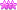elite*gold: 106The Black Market: 2/0/0 Join Date: Oct 2006 Posts: 6,045 Received Thanks: 1,160 #Moved« [Help] Dis City Mob Spawns | Ring Disapearing? »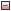Similar Threads noob question from noob player 06/03/2010 - Archlord - 14 Replies How much XP does a limpit (lv89) give at lv98 ? Because a friend of mine killed deathmute (lv79) when he was arround 89 or 90 and he only got 1000XP is this the same for the limpits? or still getting 100K xp for them at least? Kind regards and hfffffffff in game all How can i compile (Noob Question but i am noob at this) 08/04/2009 - CO2 Private Server - 6 Replies Hello all i got this problem that when i changed something in source it doesnt changes inside how do i compile ;) please help :) Hello, a short question about CR ( its not big noob question)! 02/11/2009 - Cabal Online - 0 Replies Hello, i wantet so ask in what pc langauge is cabalrider written i mean java, c++ etc. someone know? and someone know what i need to make something like a cabalrider bot self... for a other game sorry for my bad english iam just from germany i hope someone can help me ty noob question 10/16/2008 - Dekaron - 1 Replies now with the new version are u able to log into 2moons without crc bypass?

All times are GMT +2. The time now is 19:59.

 -- elitepvpers ---- Mobile Skin -- English (US) -- German (DE)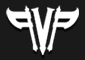Support | Contact Us | FAQ | Advertising | Privacy Policy | Terms of Service | Abuse Copyright ©2019 elitepvpers All Rights Reserved.